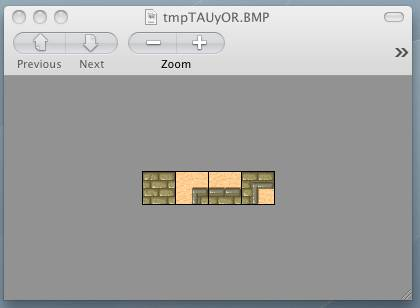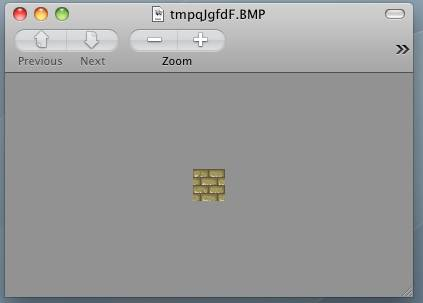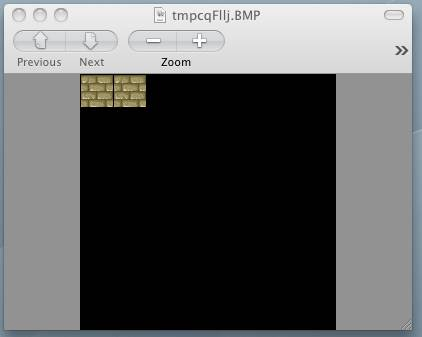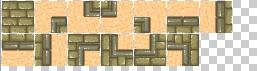# Python Tutorial: How to Generate Game Tiles with Python Imaging Library

A Python tutorial on how you can use Python Imaging Library to generate tiles for your game. By Ray Wenderlich.

Leave a rating/review
Save for later
Share

## Python Tutorial: How to Generate Game Tiles with Python Imaging Library

10 mins

The next project I’m working on involves a lot of work with Cocos2D and tile maps. And with tile maps, you’ll often have some tiles that are exactly the same, except they need to be rotated or combined with another tile in some way.

Manually rotating and laying out these tiles by hand would be very tedious and error prone. So I started looking for a way to write a script to generate images from a source set, and came across the Python Imaging Library!

In this Python tutorial, we’ll cover how to use the Python Imaging Library to write a script to read a source image, pull out each tile, and generate rotated copies of each tile into a final sprite sheet image.

It is helpful, but not 100% necessary, to have some Python knowledge for this tutorial. Also, you may wish to check out my tutorial series on How To Make A Tile Based Game with Cocos2D if you’re interested in learning how to make a tile-based game with tiles like these on the iPhone.

Ok, so let’s get started!

## Hello, Python Imaging Library!

The first thing you’ll need to do is install the Python Imaging Library (PIL for short) on your machine.

Sadly, I installed PIL on my machine some tile ago and didn’t keep good notes on the steps I took to install it, so you’re on your own!

A good place to get started is the PIL home page, where you can download the source for PIL, or instructions from Google on installing PIL on Mac OS X.

Once you have PIL installed, download a a sample image I made by pulling out a few tiles from the desert map tile set that comes with the Tiled map editor.

Then create a file named “generateTiles.py” in the same directory as the image:

```from PIL import Image

image = Image.open("DesertWall.jpg")
image.show()
```

Here we import the Image class from PIL, and use the “open” method to return an Image object. We then use the show method on the image to display a preview to our screen!

Run the script (by running “python generateTiles.py” from a Terminal) and if all works well, you should see the image pop up to the screen!Ok, so let’s modify our script to use a class to store the image, and have a helper function to read out a particular tile.

PIL makes this easy with a function named “crop” that you can call on an image. You simply pass in the coordinates for the corner of the box, and it returns another image object to you.

Replace the contents of generateTiles.py with the following:

```from PIL import Image

def __init__(self, imageName, tileSize):
self.spritesheet = Image.open(imageName)
self.tileSize = tileSize
self.margin = 1

def getTile(self, tileX, tileY):
posX = (self.tileSize * tileX) + (self.margin * (tileX + 1))
posY = (self.tileSize * tileY) + (self.margin * (tileY + 1))
box = (posX, posY, posX + self.tileSize, posY + self.tileSize)
return self.spritesheet.crop(box)

tile1.show()
```

Here we just refactor our code into a class, and we store a reference to the sprite sheet, tile size, and margin when we start up.

In the getTile method we use some math to figure out the x, y coordinates for a tile coordinate. We then figure out the coordinates for all four edges, and call crop to return a result image, which we then show.

Give it a try, and if all works well you should see the first tile show up:## Writing Tiles

Now let’s extend our code so that we can write tiles out to an output sprite sheet.

To do this in PIL, first we need to create an empty image with the Image.new() method. This lets us specify the size of the sprite sheet, and a default color (we’ll use transparent).

Once we have the empty image, we can paste an image into it by using the “paste” method.

Let’s see this in action! Add the following class right above the SpriteSheetReader class:

```class SpriteSheetWriter:

def __init__(self, tileSize, spriteSheetSize):
self.tileSize = tileSize
self.spriteSheetSize = spriteSheetSize
self.spritesheet = Image.new("RGBA", (self.spriteSheetSize, self.spriteSheetSize), (0,0,0,0))
self.tileX = 0
self.tileY = 0
self.margin = 1

def getCurPos(self):
self.posX = (self.tileSize * self.tileX) + (self.margin * (self.tileX + 1))
self.posY = (self.tileSize * self.tileY) + (self.margin * (self.tileY + 1))
if (self.posX + self.tileSize > self.spriteSheetSize):
self.tileX = 0
self.tileY = self.tileY + 1
self.getCurPos()
if (self.posY + self.tileSize > self.spriteSheetSize):
raise Exception('Image does not fit within spritesheet!')

self.getCurPos()
destBox = (self.posX, self.posY, self.posX + image.size, self.posY + image.size)
self.spritesheet.paste(image, destBox)
self.tileX = self.tileX + 1

def show(self):
self.spritesheet.show()
```

The init method here creates an empty image of the specified sprite sheet dimensions. It initializes the current tile position to 0,0.

In getCurPos, it calculates the x,y coordinates for the current tile. It has the smarts to check to see if the tile would overlap the edge of the sprite sheet and advance the y coordinate if so.

In addImage, we simply calculate the coordinates for the four corners where we want to paste the image, then call the paste method. Finally we advance the tile x coordinate to the next tile.

You can test this out by modifying the code at the bottom of the file to the following:

```reader = SpriteSheetReader("DesertWall.jpg", 32)
writer = SpriteSheetWriter(32, 256)
writer.show()
```

Run the script and you should see the following!## Rotating Images

We’re making good progress so far, but what we really wanted to do was automatically rotate our images into the sprite sheet!

Luckily this is insanely easy with PIL – we can just use the rotate method! Add the following two functions to SpriteSheetWriter:

```def addImageAndRotations(self, image):
image = image.rotate(90)
image = image.rotate(90)
image = image.rotate(90)

def save(self, imageName):
self.spritesheet.save(imageName)
```

Here we save four copies of the passed in image: the original image, and then we rotate/save 3 more times.

We also add in a method to save this off to disk so we can examine our beautiful result.

Test it out by modifying the code at the bottom to the following:

```reader = SpriteSheetReader("DesertWall.jpg", 32)
writer = SpriteSheetWriter(32, 256)
writer.show()
writer.save("DesertWallOut.jpg")
```

Run the script and open up the resulting PNG, and you should see the following:Note that when you call show() it will often show transparent regions as black, but they are really transparent when you save the image don’t worry :]

## Where To Go From Here?

Here’s a copy of the Python script using PIL we’ve developed in the above Python tutorial.

At this point you should know the basics of using PIL for saving yourself time for monotonous image manipulation like this.

It’s definitely come in handy for me for my latest Cocos2D tile-based project! Speaking of which, if you’re interested in following the development of the project, you can follow @razeware on Twitter!).

If any of you have used PIL for your projects, please comment below! :]Ray Wenderlich

Author